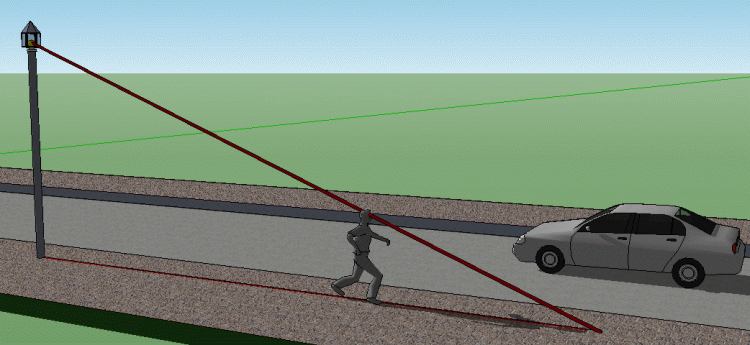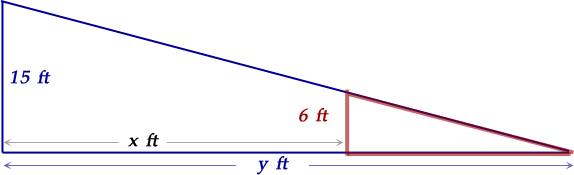SEARCH HOMEMath Central Quandaries & QueriesQuestion from Casey, a student: A street light is mounted at the top of a 15ft pole. A man 6ft tall walks away from the pole at a rate of 5ft per second. How fast is the tip of his shadow moving when he is 40ft from the pole?Hi Casey.

As the man goes farther from the street light, his shadow grows.You can see from the diagram and the extra lines I drew on it, that you have two similar right triangles here:So, y/(y-x) = 15/6 and you can solve for y: y=5x/3.

Now differentiate both sides of this equation with respect to time t, then substitute in what you know for the values of the variables. Since you want the speed of the shadow, you want to find out what dy/dt equals.

Note that the answer you get will be a bit counterintuitive, because we expect the speed of the shadow to be changing, but actually the speed of the shadow is constant (albeit faster) than the constant speed of the pedestrian regardless of distance from the light.

Cheers,
Stephen La Rocque and Penny Nom.Math Central is supported by the University of Regina and The Pacific Institute for the Mathematical Sciences.